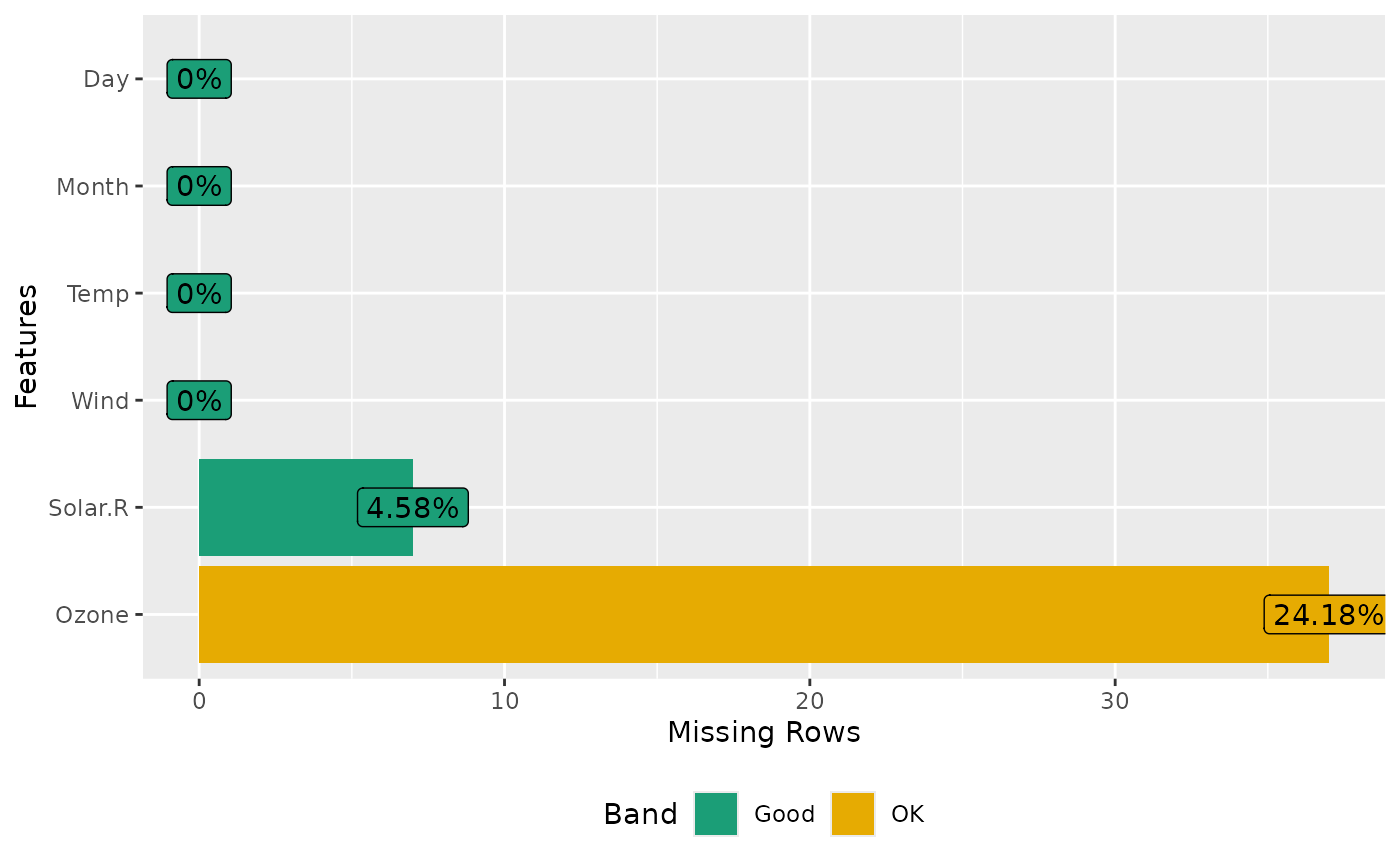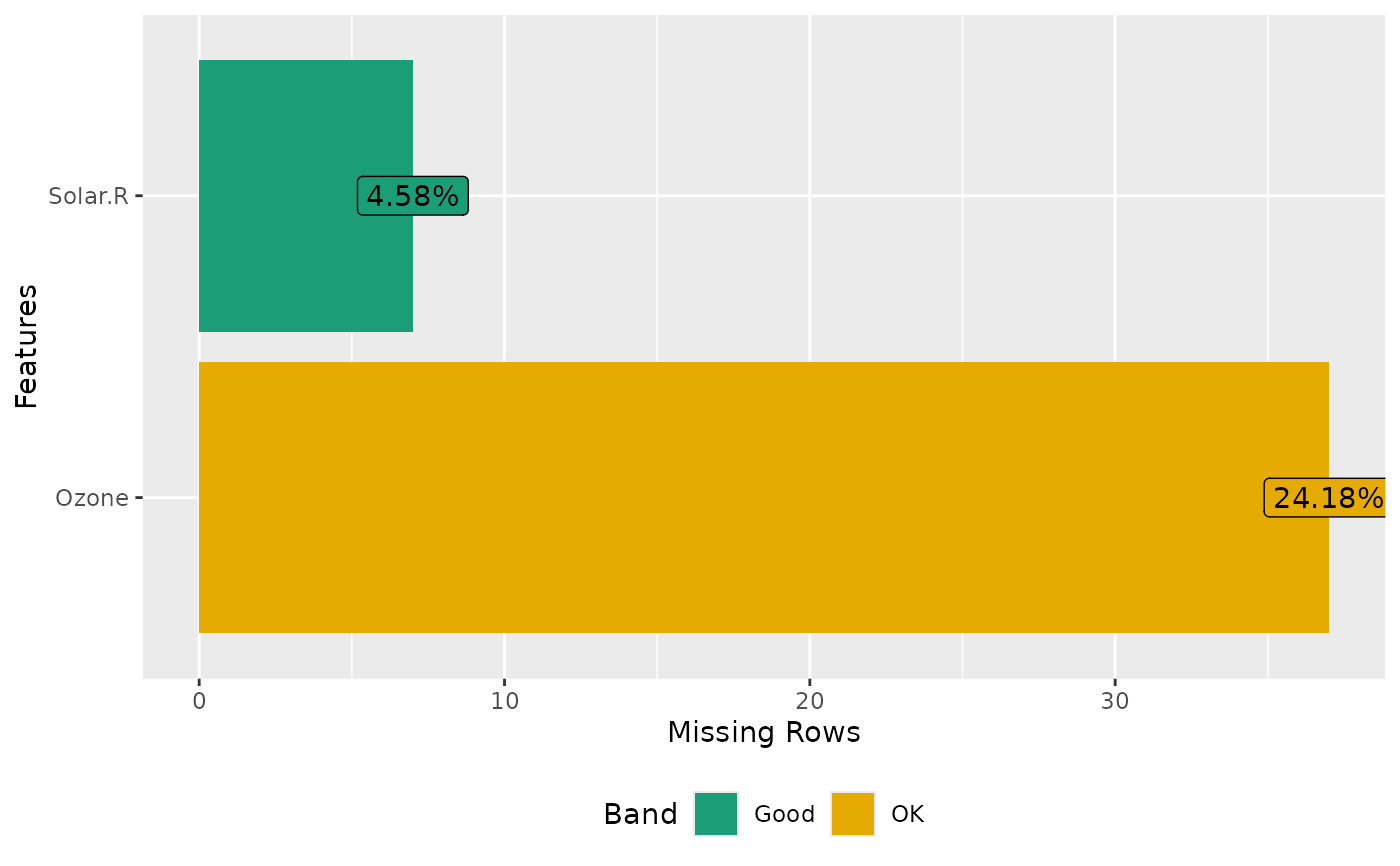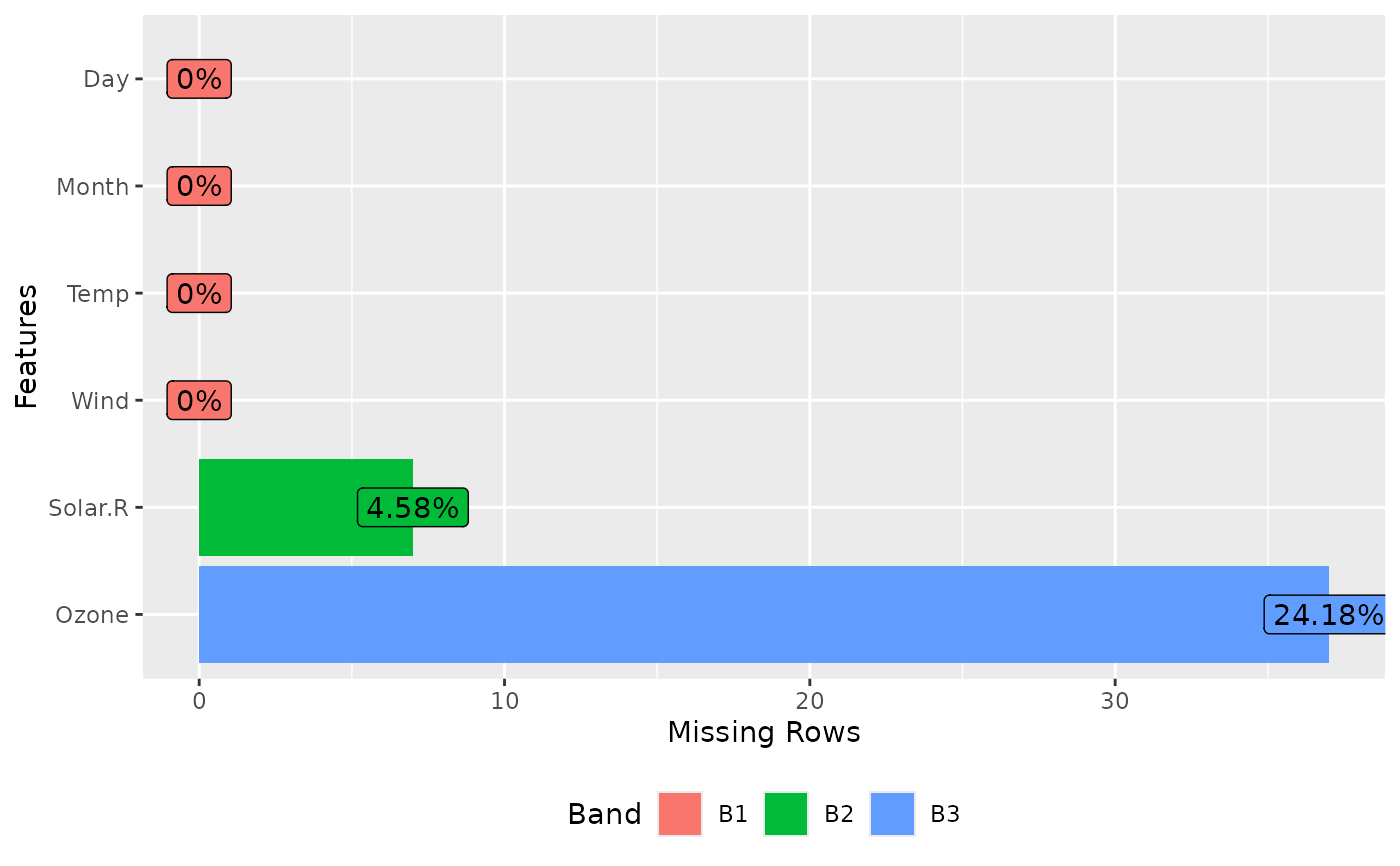This function returns and plots frequency of missing values for each feature.

plot_missing(data, group = list(Good = 0.05, OK = 0.4, Bad = 0.8, Remove
= 1), geom_label_args = list(), title = NULL,
ggtheme = theme_gray(), theme_config = list(legend.position =
c("bottom")))

## Arguments

data input data missing profile band taking a list of group name and group upper bounds. Default is list("Good" = 0.05, "OK" = 0.4, "Bad" = 0.8, "Remove" = 1). a list of other arguments to geom_label plot title complete ggplot2 themes. The default is theme_gray. a list of configurations to be passed to theme.

## Value

invisibly return the ggplot object

plot_missing(airquality)plot_missing(airquality, group = list("B1" = 0, "B2" = 0.06, "B3" = 1))plot_missing(airquality, geom_label_args = list("size" = 2, "label.padding" = unit(0.1, "lines")))